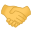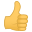# Othercount digits recursive

#### astyle

For a java only version i must dig a bit deeper. Because there is a multitude of libraries/classes.
The output of an operator in one library cannot be used as input for a function in other library etc...
I beg to differ. Most languages support 'Type conversion API'.
For example:
Java:
``````char a = "1";
char b = "2";
printf(a+b); // will output "12"
printf(atoint(a) + atoint(b)); // will output "3"
printf(atoint(a+b)); // will output "12". Figuring out why not 3 is left as an exercise for the reader.``````
Java has awareness of scope (global vs. local variables).

#### Jose

This Clojure version is interesting,
Code:
``````(def fib-seq-seq
((fn fib [a b]
(lazy-seq (cons a (fib b (+ a b)))))
0 1))
(take 30 fib-seq-seq)``````
Direct copy-paste from here:

#### Alain De Vos

Off-course. Nothing wrong with google, nor with ctrl-c , ctrl-v. I'm as much the owner of the code as i am of the clojure compiler itself.
You can't expect to link all sources, when the sources are the first results of the most basic google search, contain merely 5 lines of code, and have not a new invention.
What if the editor in wikipedia took the code from a book ?
Note : When i paste larger pieces of code & text with new ideas, i always cite the author's as form of courtesy.
Something more interesting I've read the JVM is not capable of detecting itself possible tail-call-optimisation.

#### Jose

I'm as much the owner of the code as i am of the clojure compiler itself.
No. No you are not. It's clear you have very little understanding of what you're plagiarizing.
You can't expect to link all sources...
Yes. Yes, I can. What you're doing has a name. It's called plagiarism. Passing off others' work as your own.

#### Alain De Vos

No, your wrong. Where did i put a claim to have written something myself ?
Where on that page do you see the editor of the page referencing the books he has read in order to write the article ?
Where on that page does the author claims to be the inventor of the code written ?
Please also note a wikipedia has a more "permanent" character compared to a "temporal" forum.

Here is code , i've written code, i've copied code, one can be clearer right , but only a lawyer would fall for it ?

#### a6h

Not to hijack the thread, but I have a question for software engineers -- which I'm not. I'm wondering which one is more efficient (Big O), in C :
Counting digits using Recursion, or using Natural Logarithm Common Logarithm (base 10) library function.

#### Alain De Vos

How is a logarithm implemented ?
It could be f(x)=a+bx+cx²+dx^3 , and then you have to do the additions and multiplications. O(nlog(n)) ? Probably the least efficient.
I would go for something like a constant-stack-size tail-optimized recursion (with accumulator). O(n)

PS1: When numbers are stored in binary format you can do some form of binary search towards the first "one-bit".
PS2: When numbers are stored in "exponential/double/float format" , the time is clearly O(1).

•a6h

#### astyle

I think that what Jose is quibbling on a bit of a gray area... there's enough code to demonstrate an idea from the language, to demonstrate an understanding of the concepts - but not enough to do anything particularly useful on its own. I sometimes post code snippets to show an understanding of an API - there's lots of places that code could have come from. I never claim to have invented an idea. I sometimes do quote where I got it from, if there's a debate on the correct way to do something.

#### Alain De Vos

x=123
y=log_10(123)=ln(123)/ln(10)
floor(y)+1=2+1=3
i.e. 123 has 3 digits.

•covacat

#### astyle

How is a logarithm implemented ?
It could be f(x)=a+bx+cx²+dx^3 , and then you have to do the additions and multiplications. O(nlog(n)) ? Probably the least efficient.
I would go for something like a constant-stack-size tail-optimized recursion (with accumulator). O(n)

PS1: When numbers are stored in binary format you can do some form of binary search towards the first "one-bit".
PS2: When numbers are stored in "exponential/double/float format" , the time is clearly O(1).
Please don't confuse Big O complexities with actual logarithmic functions. Big O complexities merely fit a curve that can be described by a logarithmic equation. But actually implementing the math formula that is a logarithmic equation...a logarithm is the inverse of the power function...so your example is mathematically incorrect.

#### covacat

log is inverse of exp, not pow
and you can use logarithm to count digits
bc -l -e 'scale=0;l(12345)/l(10) + 1'

••a6h and Alain De Vos

#### Alain De Vos

Logarithm is used alot in big-O notation and time-complexity of algorithms (eg sort).
O(n^n)>O(n!)>O(e^n)>O(n^2)>O(n.ln(n))>O(n)>O(ln(n))>O(1)
Here we want to use the big-O notation on the calculation of the logarithmic function.

For a number that is stored in C or IEEE double format you have:
x=precision * 10^ exponent.
To calculate the log of it : y=log_10(x)=log_10(precision)+exponent.
log_10(precision) and exponent can be calculated in constant time ie O(1).

Log is calculated using approximative polynomials. This involves multiplication. [Taking the exponent of the result must give an acceptable error]
For the precision of numbers stored in C/IEEE format (fixed length) log can be calculated in constant time O(1).
For numbers stored in other formats (variable length) its done somewhere between O(n^2) and O(nlog(n)) at best.
O(n^2): time to multiply two digits of n numbers in a "simple way"
O(nlog(n)): time for a FFT or a binary search (eg checking if the exponent of a new approximation for the log is closer to the original)

•a6h

#### astyle

•covacat

#### a6h

For numbers stored in other formats (variable length) its done somewhere between O(nlog(n)) & O(n^2)
O(n^2): time to multiply two digits of n numbers in a "simple way"
O(nlog(n)): time for a FFT.
Just for clarifications:
Those logs all are in base 10, not base e (natural logarithm). I've maid a mistake in my original question. I meant to say common logarithm (base 10), not natural (base e).
I corrected that.

#### astyle

Just for clarifications:
Those logs all are in base 10, not base e (natural logarithm). I've maid a mistake in my original question. I meant to say common logarithm (base 10), not natural (base e).
I corrected that.
I'd think recursion would be more efficient. C has a useful API for that. If you try to get fancy and use log equations (doesn't matter which ones) to get sidetracked, that's extra processor cycles every step of the way. Converting between a logarithmic representation of a number and an integer representation of the same number - that's extra computation that is unnecessary in most scenarios.•a6h

#### Jose

One thing that always trips me up: When CS types say "log" they don't mean either natural log (i.e., base 2.72) or base 10 log. They mean base 2 log. This is common in big-O analysis. So O(nlog(n)) means n times whatever power you have to raise 2 to get n.

#### Alain De Vos

Wrong , O(log_10(n))==O(ln_e(n)) , because in the limit when n goes to infinite the ratio remains constant and does not goes to one of the values zero or infinity.
ln is used for base e
log is used for base 10
log base 2 is hardly used expect in specific contexts. The precision of floating points ... CS ...

#### Jose

Wrong , O(log_10(n))==O(ln_e(n)) , because in the limit when n goes to infinite the ratio remains constant and does not goes to one of the values zero or infinity.
The ratio of what?
ln is used for base e
log is used for base 10
High school Algebra.
log base 2 is hardly used expect in specific contexts. The precision of floating points ...
Right. Specific contexts like "Computer Science".

•Alain De Vos

#### covacat

you're both right•Alain De Vos

#### astyle

One thing that always trips me up: When CS types say "log" they don't mean either natural log (i.e., base 2.72) or base 10 log. They mean base 2 log. This is common in big-O analysis. So O(nlog(n)) means n times whatever power you have to raise 2 to get n.
I would have to dig out my college-era textbook to dispute that. The point of 'log n' in Big O analysis is to show a class of computational complexity. A logarithmic function n log (n) has roughly the same shape as n ln (n) or any other logarithmic function - just grows VERY slowly, eventually flattening to a constant as n approaches infinity.

#### Alain De Vos

When f(x) is the complexity/time for a procedure dependent on a value x
And g(x) is the complexity/time for another procedure dependent on a value x
Then O(f(x)) and O(g(x)) only differ if :
lim x->infty [f(x)/g(x)] is zero or infinity.
If lim x->infty [f(x)/g(x)] is a rational number then the O numbers O(f(x)) & O(g(x)) are considered equal.

••a6h and Jose

#### Jose

Ah, I've heard that as you ignore constant factors in big-O notation. Since log2(x) = 1/log10(2) * log10(x), which is just 0.30103 * log10(x) the two expressions are equivalent. This is only true for big-O notation, however.

It's still easier to do big-O analysis if you remember logs are base-2 in CS.

#### a6h

One thing that always trips me up: When CS types say "log" they don't mean either natural log (i.e., base 2.72) or base 10 log
Interesting enough, whenever I think of logarithm, base e comes to my mind, and when I type (not write) it, I mix them up, and have to go back and fix my error!

#### Jose

Interesting enough, whenever I think of logarithm, base e comes to my mind, and when I type (not write) it, I mix them up, and have to go back and fix my error!
I guess I'm just old. In school "log" meant base-10 log, when they were still useful in the time before calculators. Natural log was always "ln". No other bases were explicitly taught.

••a6h and covacat

#### covacat

for us was lg for log10 and ln for natural logarithm and log was general and had to have a base specified